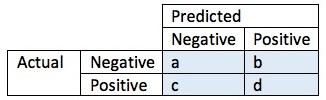# Confusion Matrix

Share on

A confusion matrix, in predictive analytics, is a two-by-two table that tells us the rate of false positives, false negatives, true positives and true negatives for a test or predictor. We can make a confusion matrix if we know both the predicted values and the true values for a sample set.

In machine learning and statistical classification, a confusion matrix is a table in which predictions are represented in columns and actual status is represented by rows. Sometimes this is reversed, with actual instances in rows and predictions in columns. The table is an extension of the confusion matrix in predictive analytics, and makes it easy to see whether mislabeling has occurred and whether the predictions are more or less correct.

A confusion matrix is also known as an error matrix, and it is a type of contingency table.

## Terminology Related to a Confusion Matrix

Suppose your confusion matrix is a simple 2 by 2 table, given by:Here a is the number of true negatives, and d the number of true positives. b is the number of false positives, and c is the number of false negatives.

• The accuracy of the prediction or test is defined as (a + d)/(a + c + d + e).
• The true positive rate is given by d/(c + d), and is also called the recall. It tells us what proportion of positive cases were correctly identified.
• The false positive rate, or proportion of negative cases (incorrectly) identified as positive, is given by b/(a + b).
• The true negative rate is a/(a + b), and represents the proportion of negative cases that were correctly identified.
• The false negative rate is c/(c + d), and tells us what proportion of positive cases were incorrectly labeled as negative.
• The proportion of the instances we correctly labeled as positive (per total positive prediction) is given by d/(b + d) and is called the precision.

## References

Hamilton, Howard J. Confusion Matrix. Course Notes for Computer Science 831: Knowledge Discovery in Databases. Retrieved from http://www2.cs.uregina.ca/~hamilton/courses/831/notes/confusion_matrix/confusion_matrix.html on August 1, 2018.
Oliver, Arnau. Confusion Matrices. Evaluation Matrices, 2008-06-17. Retrieved from http://eia.udg.edu/~aoliver/publications/tesi/node143.html on August 3, 2018.

CITE THIS AS:
Stephanie Glen. "Confusion Matrix" From StatisticsHowTo.com: Elementary Statistics for the rest of us! https://www.statisticshowto.com/confusion-matrix/
---------------------------------------------------------------------------Need help with a homework or test question? With Chegg Study, you can get step-by-step solutions to your questions from an expert in the field. Your first 30 minutes with a Chegg tutor is free!